Ex 1.4

Chapter 1 Class 11 Sets (Term 1)
Serial order wise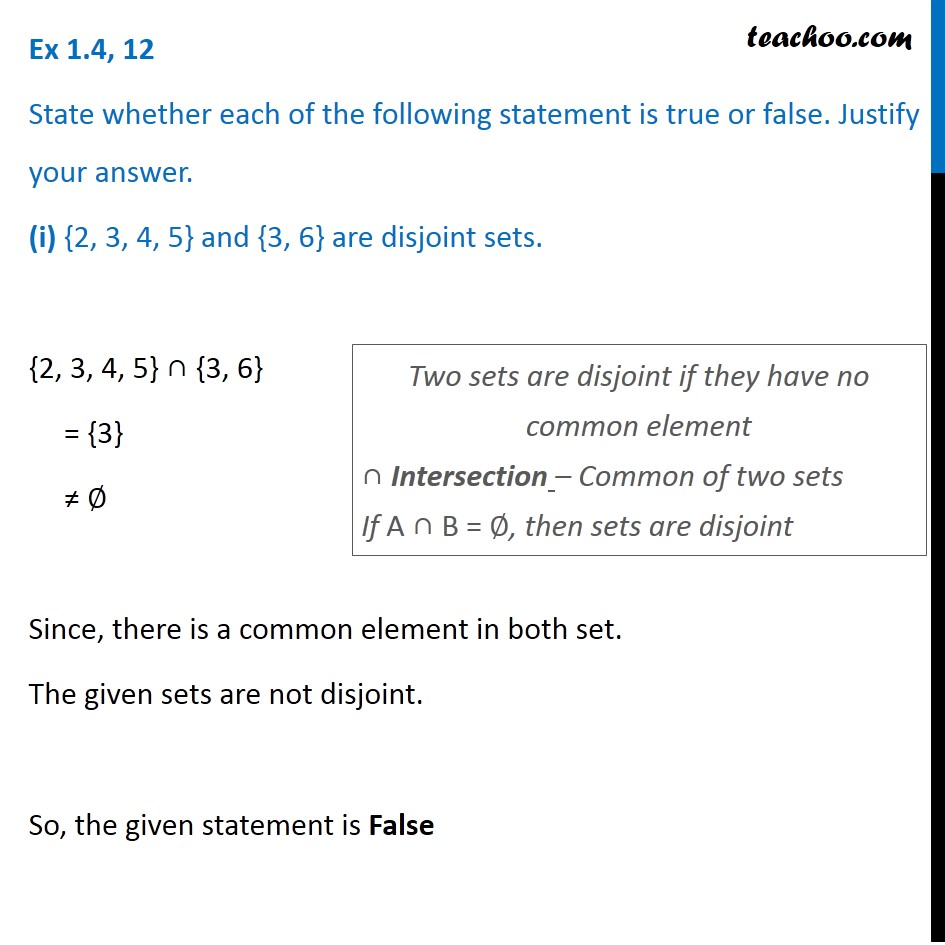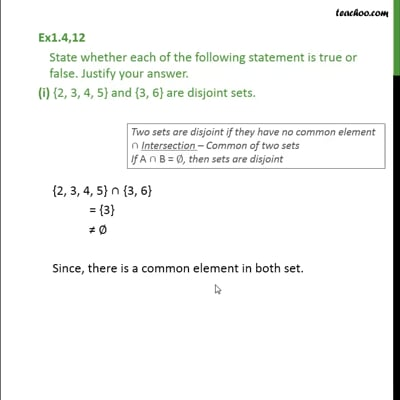This video is only available for Teachoo black users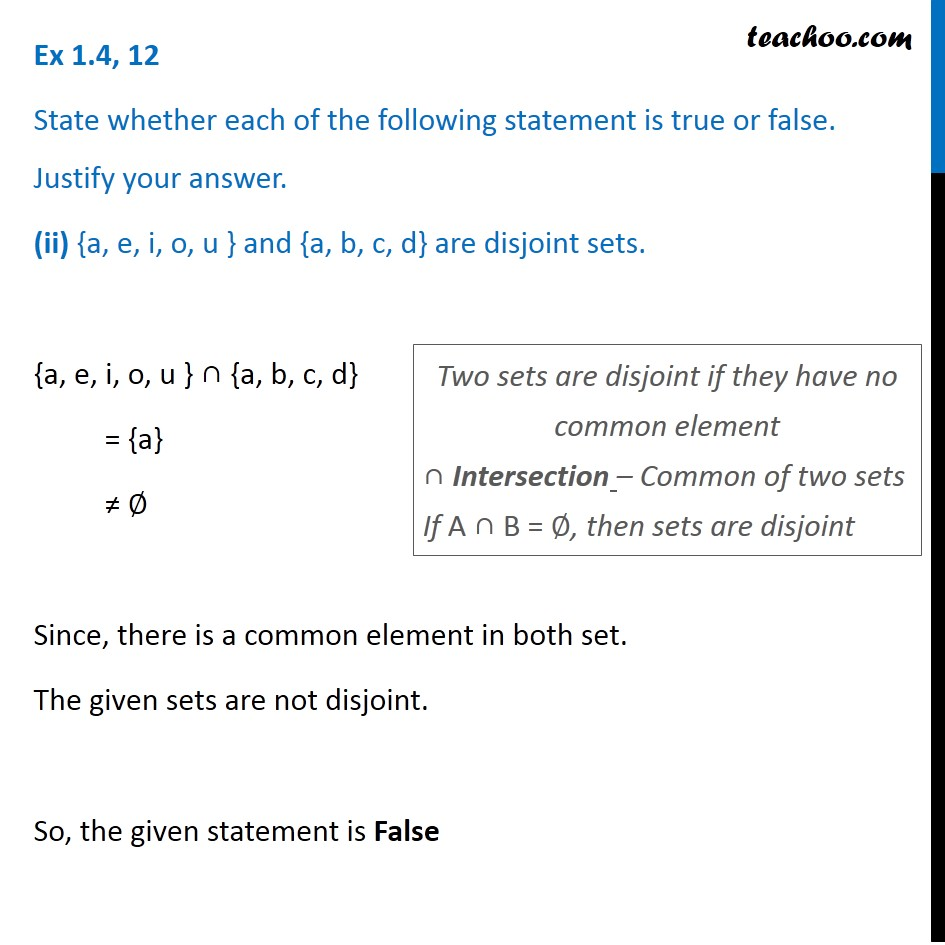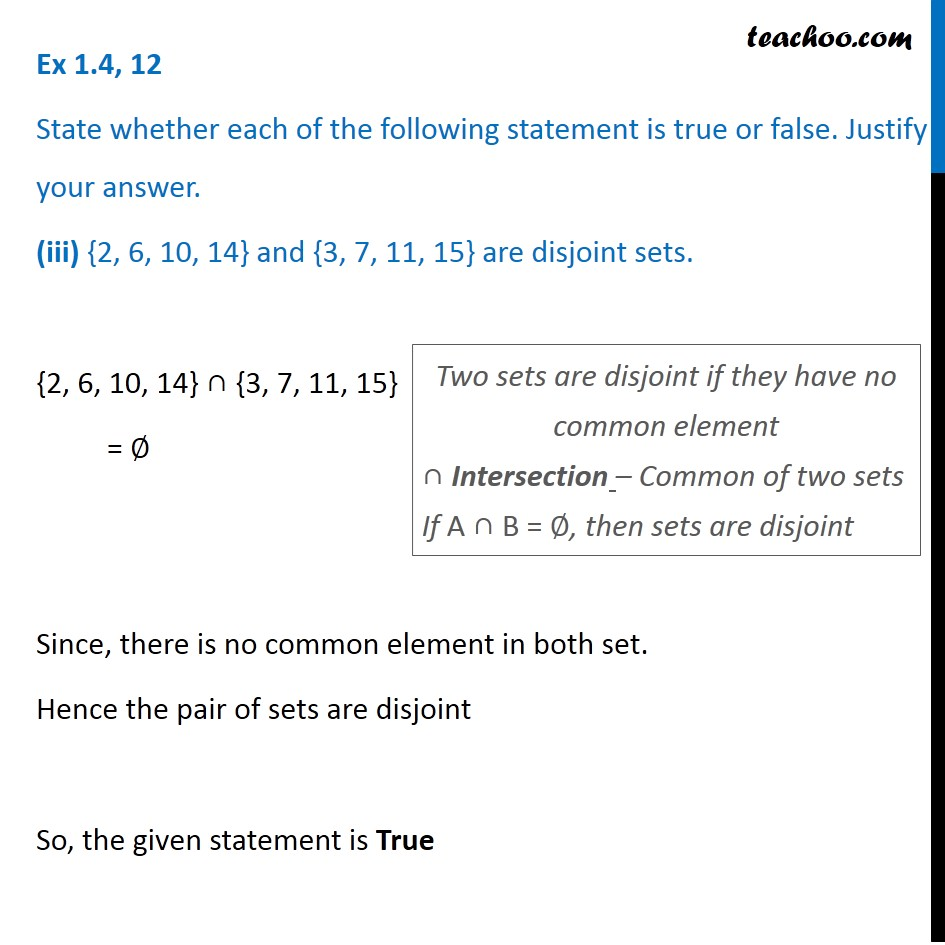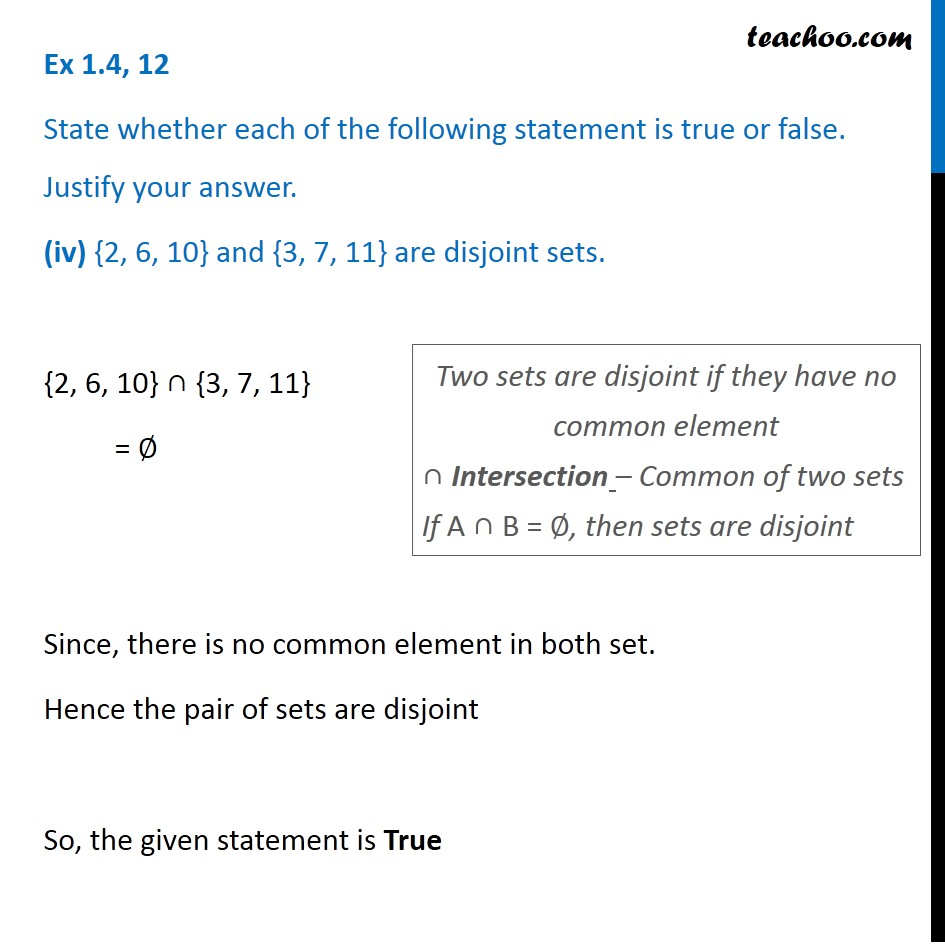### Transcript

Ex 1.4, 12 State whether each of the following statement is true or false. Justify your answer. (i) {2, 3, 4, 5} and {3, 6} are disjoint sets. {2, 3, 4, 5} ∩ {3, 6} = {3} ≠ ∅ Since, there is a common element in both set. The given sets are not disjoint. So, the given statement is False Two sets are disjoint if they have no common element ∩ Intersection – Common of two sets If A ∩ B = ∅, then sets are disjoint Ex 1.4, 12 State whether each of the following statement is true or false. Justify your answer. (ii) {a, e, i, o, u } and {a, b, c, d} are disjoint sets. {a, e, i, o, u } ∩ {a, b, c, d} = {a} ≠ ∅ Since, there is a common element in both set. The given sets are not disjoint. So, the given statement is False Two sets are disjoint if they have no common element ∩ Intersection – Common of two sets If A ∩ B = ∅, then sets are disjoint Ex 1.4, 12 State whether each of the following statement is true or false. Justify your answer. (iii) {2, 6, 10, 14} and {3, 7, 11, 15} are disjoint sets. {2, 6, 10, 14} ∩ {3, 7, 11, 15} = ∅ Since, there is no common element in both set. Hence the pair of sets are disjoint So, the given statement is True Two sets are disjoint if they have no common element ∩ Intersection – Common of two sets If A ∩ B = ∅, then sets are disjoint Ex 1.4, 12 State whether each of the following statement is true or false. Justify your answer. (iv) {2, 6, 10} and {3, 7, 11} are disjoint sets. {2, 6, 10} ∩ {3, 7, 11} = ∅ Since, there is no common element in both set. Hence the pair of sets are disjoint So, the given statement is True Two sets are disjoint if they have no common element ∩ Intersection – Common of two sets If A ∩ B = ∅, then sets are disjoint